# Moderator Analysis with a Dichotomous Moderator using SPSS Statistics

## Introduction

A moderator analysis is used to determine whether the relationship between two variables depends on (is moderated by) the value of a third variable. This relationship is commonly between: (a) a continuous dependent variable and continuous independent variable, which is modified by a dichotomous moderator variable; (b) a continuous dependent variable and continuous independent variable, which is modified by a polytomous moderator variable; or (c) a continuous dependent variable and continuous independent variable, which is modified by a continuous moderator variable. In this guide, we focus on (a); namely, the relationship between a continuous dependent variable and continuous independent variable, which is modified by a dichotomous moderator variable.

We use the standard method of determining whether a moderating effect exists, which entails the addition of an (linear) interaction term in a multiple regression model. For this reason, you might often hear this type of analysis being referred to as a moderated multiple regression or as its abbreviation, MMR (e.g., Aguinis, 2004). Indeed, a moderator analysis is really just a multiple regression equation with an interaction term. What makes it a moderator analysis is the theory and subsequent hypotheses that surround this statistical test (e.g., Aguinis, 2004; Jaccard & Turrisi, 2003; Jose, 2013).

For example, a moderator analysis can be used to determine whether the relationship between HDL cholesterol and amount of exercise performed per week is different for normal weight and obese participants (i.e., the continuous dependent variable is "HDL cholesterol", the continuous independent variable is "amount of exercise performed per week" and the dichotomous moderator variable is "body composition", consisting of two groups: "normal weight" and "obese")? If it is, body composition (i.e., the dichotomous moderator variable) moderates the relationship between the amount of exercise performed per week and HDL cholesterol concentration. Alternately, you could use a moderator analysis to determine whether the relationship between salary and years of education is moderated by gender (i.e., the continuous dependent variable is "salary", the continuous independent variable is "years of education" and the dichotomous moderator variable is "gender", which consists of two groups: "males" and "females"). If it is, gender (i.e., the dichotomous moderator variable) moderates the relationship between the years of education and salary.

This "quick start" guide shows you how to carry out a moderator analysis with a dichotomous moderator variable using SPSS Statistics, as well as interpret and report the results from this test. However, before we introduce you to this procedure, you need to understand the different assumptions that your data must meet in order for the moderator analysis to give you a valid result. We discuss these assumptions next.

## Assumptions

When you choose to run a moderator analysis using multiple regression, part of the process involves checking to make sure that the data you want to analyse can actually be analysed using multiple regression. You need to do this because it is only appropriate to use a moderator analysis using multiple regression if your data "passes" eight assumptions that are required for multiple regression to give you a valid result. In practice, checking for these eight assumptions just adds a little bit more time to your analysis, requiring you to click a few more buttons in SPSS Statistics when performing your analysis, as well as think a little bit more about your data, but it is not a difficult task.

Before we introduce you to these eight assumptions, do not be surprised if, when analysing your own data using SPSS Statistics, one or more of these assumptions is violated (i.e., not met). This is not uncommon when working with real-world data rather than textbook examples, which often only show you how to carry out a moderator analysis using multiple regression when everything goes well! However, don’t worry. Even when your data fails certain assumptions, there is often a solution to overcome this. First, let's take a look at these eight assumptions:

• Assumption #1: Your dependent variable should be measured on a continuous scale (i.e., it is either an interval or ratio variable). Examples of variables that meet this criterion include revision time (measured in hours), intelligence (measured using IQ score), exam performance (measured from 0 to 100), weight (measured in kg), and so forth. You can learn more about interval and ratio variables in our article: Types of Variable.
• Assumption #2: You have one independent variable, which is continuous (i.e., an interval or ratio variable) and one moderator variable that is dichotomous (i.e., a nominal variable with two groups). For examples of continuous variables, see the bullet above. Examples of dichotomous variables include gender (e.g., two groups: male and female), physical activity level (e.g., two groups: sedentary and active), body composition (e.g., two groups: normal weight and obese), and so forth. Again, you can learn more about variables in our article: Types of Variable.
• Assumption #3: You should have independence of observations (i.e., independence of residuals), which you can check using the Durbin-Watson statistic, which is a simple test to run using SPSS Statistics.
• Assumption #4: There needs to be a linear relationship between the dependent variable and the independent variable for each group of the dichotomous moderator variable. Whilst there are a number of ways to check for these linear relationships, you can create a scatterplot using SPSS Statistics, and then visually inspecting this scatterplot to check for linearity. If the relationship displayed in your scatterplot is not linear, you will have to either run a non-linear regression analysis or "transform" your data, which you can do using SPSS Statistics. In our enhanced moderator guide, we show you how to create and interpret a scatterplot to check for linearity when carrying out a moderator analysis using SPSS Statistics and some of the options available to you when you do not have linearity.
• Assumption #5: Your data needs to show homoscedasticity, which is when the error variances are the same for all combinations of independent and moderator variables. We explain more about what this means and how to assess the homoscedasticity of your data in our enhanced moderator analysis guide. When you analyse your own data, you will need to plot the studentized residuals against the unstandardized predicted values for both groups of the moderator variable. In our enhanced moderator analysis guide, we explain: (a) how to test for homoscedasticity using SPSS Statistics; (b) some of the things you will need to consider when interpreting your data; and (c) possible ways to continue with your analysis if your data fails to meet this assumption.
• Assumption #6: Your data must not show multicollinearity, which occurs when you have two or more independent variables that are highly correlated with each other. This leads to problems with understanding which independent variable contributes to the variance explained in the dependent variable, as well as technical issues in calculating a multiple regression model. Therefore, in our enhanced moderator analysis guide, we show you: (a) how to use SPSS Statistics to detect for multicollinearity through an inspection of correlation coefficients and Tolerance/VIF values; and (b) how to interpret these correlation coefficients and Tolerance/VIF values so that you can determine whether your data meets or violates this assumption.
• Assumption #7: There should be no significant outliers, high leverage points or highly influential points. Outliers, leverage and influential points are different terms used to represent observations in your data set that are in some way unusual when you wish to perform a moderator analysis. These different classifications of unusual points reflect the different impact they have on the moderated multiple regression. An observation can be classified as more than one type of unusual point. However, all these points can have a very negative effect on the regression equation that is used to analyse this type of moderator analysis. This can change the output that SPSS Statistics produces and reduce the accuracy of your results as well as the statistical significance. Fortunately, when using SPSS Statistics you can detect possible outliers, high leverage points and highly influential points. In our enhanced moderator analysis guide, we: (1) show you how to detect outliers using "studentized deleted residuals" and discuss some of the options you have in order to deal with outliers; (2) check for leverage points using SPSS Statistics, and discuss what you should do if you have any; and (3) check for influential points in SPSS Statistics using a measure of influence known as Cook's Distance, before presenting some practical approaches in SPSS Statistics to deal with any influential points you might have.
• Assumption #8: Finally, you need to check that the residuals (errors) are approximately normally distributed. Methods to do this can be based either on graphical or numerical methods. In our enhanced moderator analysis guide, we: (a) show you how to check this assumption using the Shapiro-Wilk test for normality using SPSS Statistics; (b) explain how to interpret the result; and (c) provide possible options if your data fails to meet this assumption.

You can check assumptions #3, #4, #5, #6, #7 and #8 using SPSS Statistics. Assumptions #1 and #2 should be checked first, before moving onto assumptions #3, #4, #5, #6, #7 and #8. Just remember that if you do not run the statistical tests on these assumptions correctly, the results you get when running the moderator analysis might not be valid. This is why we dedicate a number of sections of our enhanced moderator analysis guide to help you get this right. You can find out about our enhanced content as a whole here, or more specifically, learn how we help with testing assumptions here. Alternately, you can access the enhanced moderator analysis guide now by subscribing to the site here.

In the section, Procedure, we illustrate the SPSS Statistics procedure to perform a moderator analysis assuming that no assumptions have been violated. First, we introduce the example that is used in this guide.## Example

Cholesterol has a reputation for generally being bad and being a reason for getting heart disease. However, a particular type of cholesterol called high-density lipoprotein cholesterol (HDL cholesterol, for short) is linked to good heart health. The higher the concentration of HDL cholesterol in the blood the better.

It is known that exercise can increase HDL cholesterol concentration. However, there is thought to be a complex interplay between exercise and body fat. As such, although it is known that higher levels of physical activity are associated with higher concentrations of HDL cholesterol, a researcher wants to understand whether this relationship is similar in normal weight and obese individuals (as determined by their BMI [Body Mass Index], which is a way to assess whether individuals are of a normal weight or obese).

As such, the researcher hypothesized that individuals with higher levels of physical activity would have higher concentrations of HDL cholesterol, but that this relationship would be different for individuals who are normal weight and those who are obese. In variable terms, the researcher wants to know whether body_composition statistically significantly moderates the relationship between physical_activity and HDL.

## Setup in SPSS Statistics

In SPSS Statistics, we created three variables: (1) HDL, which is the HDL cholesterol concentration; (2) physical_activity, which is the participant's level of physical activity measured in the number of minutes of exercise performed per week; (3) body_composition, which is the participant's body composition (i.e., normal weight or obese). However, the moderator variable, body_composition, cannot simple be entered into a multiple regression equation. It first needs to be "converted" into a dummy variable. What this means and how to do it is explained in our enhanced moderator analysis guide. In this guide we name the dummy variable, normal. In addition, an interaction term has to be created between the independent and moderator variables, which we will call pa_x_normal (N.B., "pa" stands for the independent variable, "physical activity", "_x_" stands for multiplication and "normal" reflects our moderator variable). Again, why and how to do this is explained in our enhanced moderator analysis guide. You can learn about our enhanced data setup content here.

## Test Procedure in SPSS Statistics

The 11 steps below show you how to run a moderator analysis in SPSS Statistics when none of the eight assumptions in the previous section, Assumptions, have been violated. At the end of these 11 steps, we show you how to interpret the results from your moderator analysis. If you are looking for help to make sure your data meets assumptions #3, #4, #5, #6, #7 and #8, which are required when performing moderator analysis and can be tested using SPSS Statistics, you can access the enhanced moderator analysis guide by subscribing to the site here.

• Click Analyze > Regression > Linear... on the main menu, as shown below: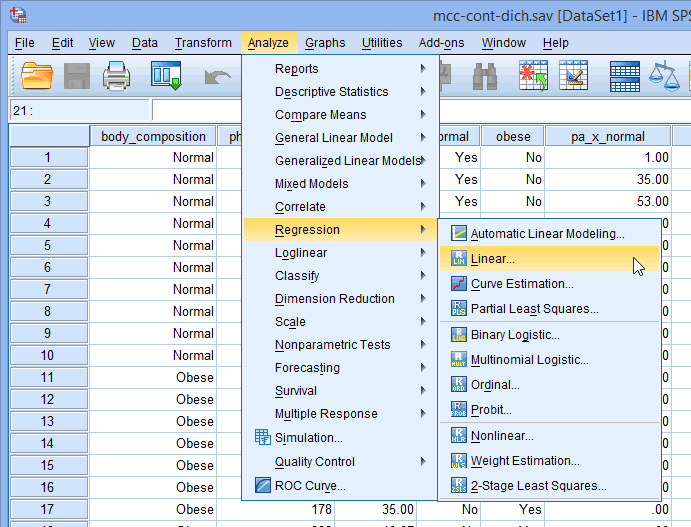Published with written permission from SPSS Statistics, IBM Corporation.

You will be presented with the Linear Regression dialogue box, as shown below: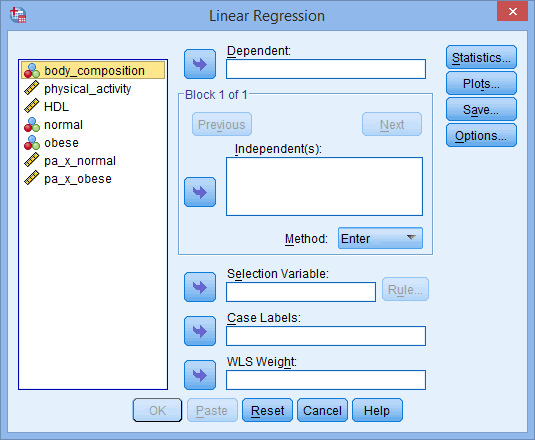Published with written permission from SPSS Statistics, IBM Corporation.

• Transfer the dependent variable, HDL, into the Dependent: box and then transfer the independent variable, physical_activity, and the dummy variable, normal, into the Independent(s): box using the appropriate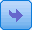buttons. You will end up with a screen similar to the one below: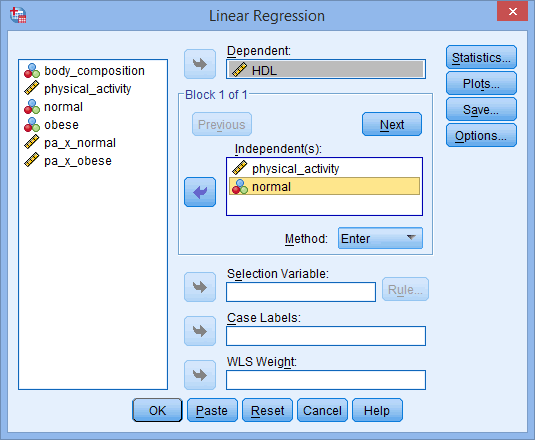Published with written permission from SPSS Statistics, IBM Corporation.

Note: The independent and dummy variables will form what will be called Model 1 in the results section generated by this procedure. Note that, at the moment, you have not transferred the interaction term (i.e., pa_x_normal).

• Click the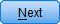button. You will be presented with the following screen: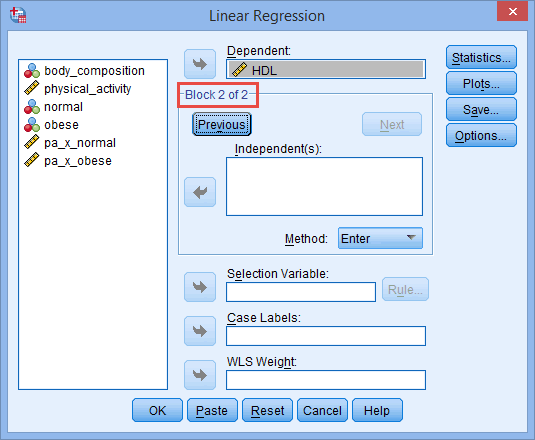Published with written permission from SPSS Statistics, IBM Corporation.

Note: Notice that the area in which the Independent(s): box resides has changed from –Block 1 of 1– to –Block 2 of 2– (as highlighted above). This explains why it looks as though your variables have disappeared from the Independent(s): box. They haven't, they are just located in –Block 1 of 2–, which can be reached by clicking on the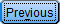button.

The Method: option needs to be kept at the default value, which is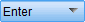. If, for whatever reason,is not selected, you need to change Method: back to.

• Transfer the interaction term (i.e., pa_x_normal) into the Independent(s): box using the appropriatebutton, as shown below: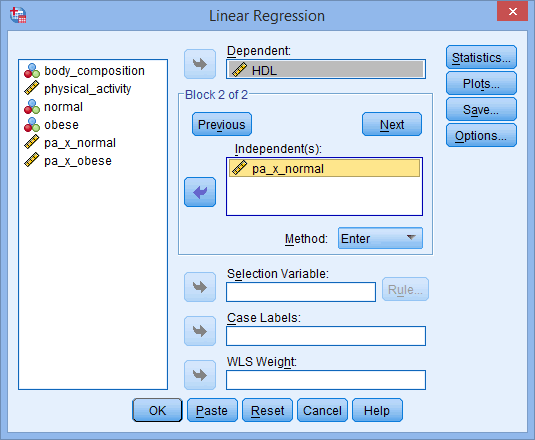Published with written permission from SPSS Statistics, IBM Corporation.

Explanation: By transferring the pa_x_normal interaction term, you are testing to see if the addition of this interaction term to the existing regression model (i.e., the model that contains only the independent and dummy variables, physical_activity and normal) improves the prediction of HDL. This will also allow you to determine whether the interaction term is statistically significant. This regression model with all three variables included in the equation – physical_activity, normal and pa_x_normal – will be called Model 2 in the results generated by this procedure. Therefore, the effect of the addition of the interaction term will be the difference between Model 1 and Model 2.

• Click the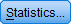button. You will be presented with the Linear Regression: Statistics dialogue box, as shown below: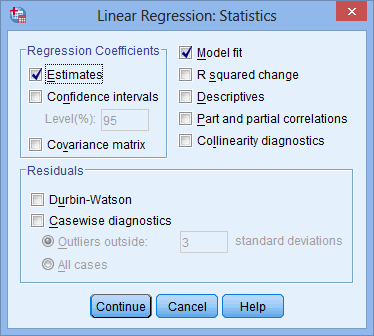Published with written permission from SPSS Statistics, IBM Corporation.

• Select the Confidence intervals option in the –Regression Coefficients– area and the R squared change and Collinearity diagnostics options. Leave the default options checked. You will be presented with the following screen: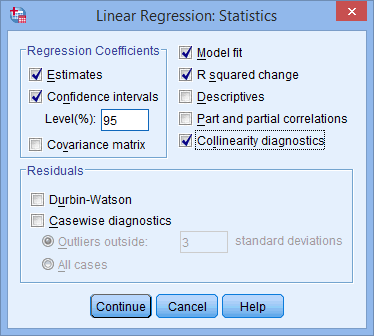Published with written permission from SPSS Statistics, IBM Corporation.

Explanation: You will use the R squared change option to determine the effect of the addition of the interaction term to the model (i.e., whether there is a moderation effect).

• Click the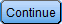button. You will be returned to the Linear Regression dialogue box.

• Click the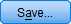button and you will be presented with the Linear Regression: Save dialogue box, as shown below: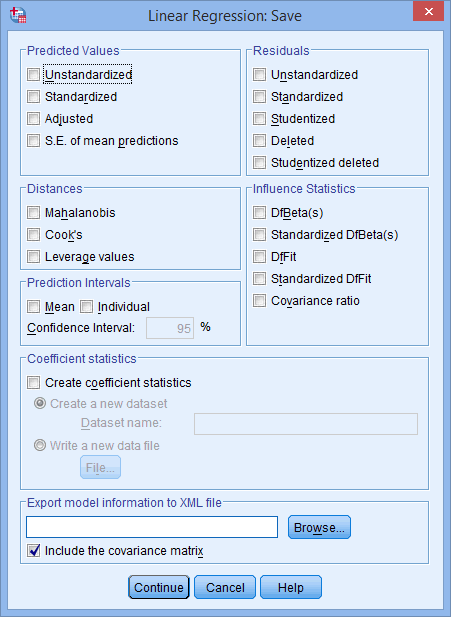Published with written permission from SPSS Statistics, IBM Corporation.

• Select Unstandardized in the –Predicted Values– area, Studentized and Studentized deleted in the –Residuals– area, and Cook's and Leverage values in the –Distances– area, as shown below: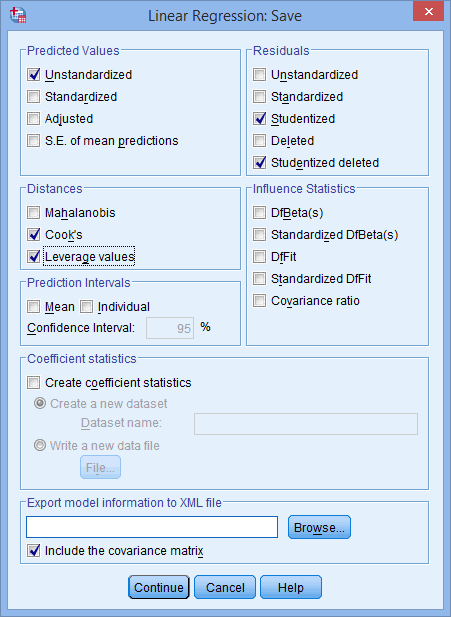Published with written permission from SPSS Statistics, IBM Corporation.

• Click thebutton. You will be returned to the Linear Regression dialogue box.

• Click the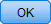button. This will generate the results.

Join the 10,000s of students, academics and professionals who rely on Laerd Statistics.

## Interpreting and Reporting the Output of the Moderator Analysis

SPSS Statistics will generate quite a few tables of output for a moderator analysis. In this section, we show you one of the tables you can use to determine whether body composition is moderating the relationship between physical activity and HDL cholesterol concentration, assuming that no assumptions have been violated. A complete explanation of the output you have to interpret when checking your data for the eight assumptions required to carry out a moderator analysis is provided in our enhanced guide. However, in this "quick start" guide, we focus only on one of the tables you can use to understand your moderator results, assuming that your data has already met these eight assumptions.

Therefore, to understand whether you have a moderator effect, you need to interpret the Model Summary table because this provides the change in R2 measure (within the "Change Statistics" columns for "Model 2"), which we can use to determine the statistical significance of the interaction term and, subsequently, whether body composition moderates the effect of physical activity on HDL cholesterol concentration, as highlighted below: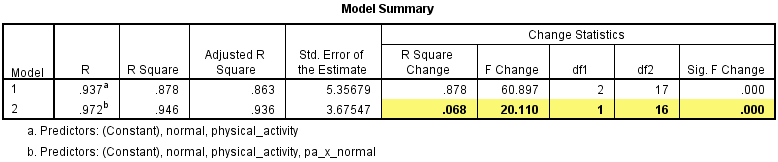Published with written permission from SPSS Statistics, IBM Corporation.

The first column highlighted, "R Square Change", shows the increase in variation explained by the addition of the interaction term (i.e., the change in R2). You can see that the change in R2 is reported as .068, which is a proportion. More usually, this measure is reported as a percentage so we can say that the change in R2 is 6.8% (i.e., .068 x 100 = 6.8%), which is the percentage increase in the variation explained by the addition of the interaction term. We can also see that this increase is statistically significant (p < .0005), a result we obtain from the "Sig. F Change" column (remembering that, in SPSS Statistics, a statistical significance value of .000 does not mean zero, but p < .0005). We can conclude that body composition does moderate the relationship between physical activity and HDL cholesterol concentration.

The method we used above is one of two available to determine whether you have a statistically significant moderator effect. The other uses the statistical significance of the interaction term. This latter method also provides valuable information on the difference between the two groups of the moderator in their relationship between the independent and dependent variable. How to understand and interpret the interaction term is provided in our enhanced moderator analysis guide.

If we wanted to report the moderated multiple regression equation, we could do so by determining the coefficient values from the "B" column in the Coefficients table, as highlighted below: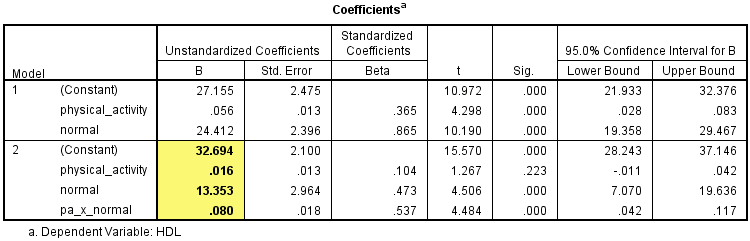Published with written permission from SPSS Statistics, IBM Corporation.

Using the values obtained above, you could report the regression equation as follows:

HDL = 32.694 + (0.016 x physical_activity) + (13.353 x normal) + (0.080 x pa_x_normal)

A fuller understanding of the equation above is provided in our enhanced moderator analysis guide.

###### SPSS Statistics

Once you have determined whether you have a statistically significant interaction, you can follow up with post hoc probing. One common approach is to consider the simple regression lines (aka simple regression slopes). The two simple regression slopes are shown in the diagram below: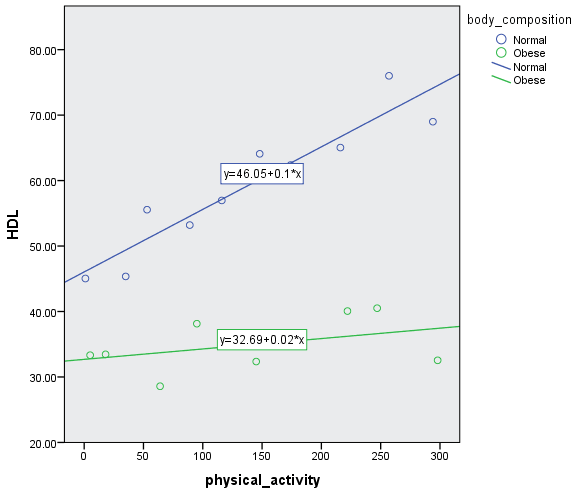Published with written permission from SPSS Statistics, IBM Corporation.

You can use follow up tests to determine whether these simple regression slopes are statistically significant. How to do this and interpret and report the results is presented in our enhanced moderator analysis guide. We also show you how to write up the results from your assumptions tests and moderator analysis output if you need to report this in a dissertation/thesis, assignment or research report. We do this using the Harvard and APA styles. You can view the enhanced moderator analysis guide by subscribing to the site here.

## References

A more extensive reference and bibliography is provided in the enhanced moderator guide.

Aguinis, H. (2004). Regression analysis for categorical moderators. New York, NY: Guilford Press.

Jaccard, J., & Turrisi, R. (2003). Interaction effects in multiple regression (2nd ed.). Thousand Oaks, CA: Sage Publications.

Jose, P. E. (2013). Doing statistical mediation & moderation. New York, NY: Guilford Press.

Join the 10,000s of students, academics and professionals who rely on Laerd Statistics.